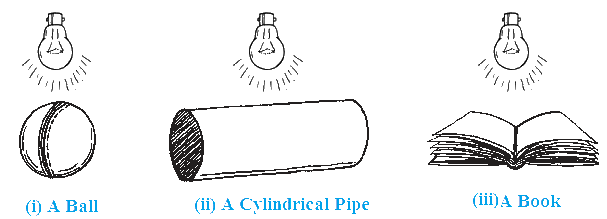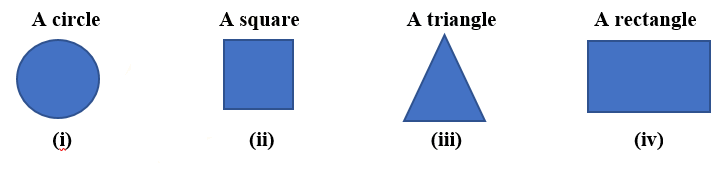# Exercise 15.4 Visualizing Solid Shapes NCERT Solutions Class 7

## Chapter 15 Ex.15.4 Question 1

A bulb is kept burning just right above the following solids. Name the shape of the shadows obtained in each case. Attempt to give a rough sketch of the shadow. (You may try to experiment first and then answer these questions).### Solution

What is known?

Some solids

What is unknown?

Shape of the shadow obtain in each case.

Reasoning:

A spherical object gives a circular shape shadow.

A cylindrical object gives a shadow of a line.

A cuboidal object gives a rectangular shape shadow.

Steps:

(i) A ball will give the shadow of a circle when tourch is above the ball.

(ii) A cylindrical pipe  will give the shadow of a line.

(iii) A book will give image of a rectangle.

## Chapter 15 Ex.15.4 Question 2

Here are the shadows of some $$3-D$$ objects, when seen under the lamp of an overhead projector. Identify the solid(s) that match each shadow. (There may be multiple answers for these!)### Solution

What is known?

Some shadow of solid shapes

What is unknown?

Solid shapes for each shadow

Reasoning:

A spherical object gives a circular shape shadow.

A cylindrical object gives a shadow of a line.

A cubical object gives a square shape shadow.

A cuboidal object gives a rectangular shape shadow.

A conical object gives a triangular shadow.

Steps:

Solids will be:

(i) A ball or sphere will give shadow of a circle.

(ii) A cube shape object like dice will give shadow of a square.

(iii) An ice- cream cone or a birthday cap will give the shadow of triangle.

(iv) A book or a cuboid shape object will give shadow of a rectangle.

## Chapter 15 Ex.15.4 Question 3

Examine if the following are true statements:

(i) The cube can cast a shadow in the shape of a rectangle.

(ii) The cube can cast a shadow in the shape of a hexagon.

### Solution

What is known?

Statements.

What is unknown?

Statement is true or not.

Reasoning:

A cubical object gives a square shape shadow when light is coming directly and it gives a rectangular shadow when light is coming diagonally.

Steps:

(i) The cube can cast a shadow in the shape of a rectangle is True because when the light is coming diagonally then it will cast shadow of rectangle.

(ii) The cube can cast a shadow in the shape of a hexagon is false as in either case cube will not cast shadow of hexagon as cube can cast shadow of square or rectangle and square and rectangle both have $$4$$ sides but a hexagon has $$6$$ sides.

Instant doubt clearing with Cuemath Advanced Math Program